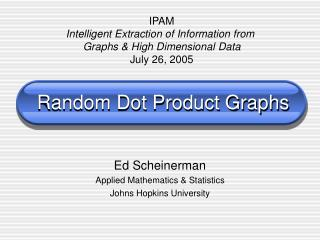DownloadDownload PresentationRandom Dot Product Graphs

# Random Dot Product Graphs

Télécharger la présentation## Random Dot Product Graphs

- - - - - - - - - - - - - - - - - - - - - - - - - - - E N D - - - - - - - - - - - - - - - - - - - - - - - - - - -
##### Presentation Transcript

1. IPAM Intelligent Extraction of Information from Graphs & High Dimensional Data July 26, 2005 Random Dot Product Graphs Ed Scheinerman Applied Mathematics & Statistics Johns Hopkins University

2. Coconspirators • Libby Beer • John Conroy (IDA) • Paul Hand (Columbia) • Miro Kraetzl (DSTO) • Christine Nickel • Carey Priebe • Kim Tucker • Stephen Young (Georgia Tech)

3. Overview • Mathematical context • Modeling networks • Random dot product model • The inverse problem

4. Mathematical Context

5. Graphs I Have Loved • Interval graphs & intersection graphs • Random graphs • Random intersection graphs • Threshold graphs & dot product graphs

6. Interval Graphs

7. Intersection Graphs {1} {1} {1,2} {2}

8. Random Graphs Erdös-Rényi style… p 1 – p Randomness is “in” the edges. Vertices are “dumb” placeholders.

9. Random Intersection Graphs • Assign random sets to vertices. • Two vertices are adjacent iff their sets intersect. • Randomness is “in” the vertices. • Edges reflect relationships between vertices.

10. Threshold Graphs 0.5 0.6 0.3 0.8

11. Dot Product Graphs [1 0] [2 0] [0 1] [1 1] Fractional intersection graphs

12. Communication Networks

13. Physical Networks Internet Telephone Power grid Local area network

14. Alice Bob Social Networks 2003-4-10 B A

15. Social Network Graphs

16. Example: Email at HP • 485 employees • 185,000 emails • Social network (who emails whom) identified 7 “communities”, validated by interviews with employees.

17. Properties of Social Networks • Clustering • Low diameter • Power law

18. Properties of Social Networks • Clustering • Low diameter • Power law b a c

19. Properties of Social Networks • Clustering • Low diameter • Power law “Six degrees of separation”

20. Properties of Social Networks • Clustering • Low diameter • Power law Degree Histogram log N(d) log d

21. Degree Histogram Example 1 2838 vertices Number of vertices degree

22. Degree Histogram Example 2 16142 vertices Number of vertices degree

23. Random Graph Models Goal: Simple and realistic random graph models of social networks.

24. Erdös-Rényi? • Low diameter! • No clustering: P[a~c]=P[a~c|a~b~c]. • No power-law degree distribution. Not a good model.

25. Model by Fan Chung et al Consider only those graphs with with all such graphs equally likely.

26. People as Vectors Sports Politics Movies Graph theory

27. Shared Interests Alice and Bob are more likely to communicate when they have more shared interests.

28. Selecting the Function

29. Selecting the Function

30. Selecting the Function

31. Random Dot Product Graphs, I

32. Generalize Erdös-Rényi

33. Generalize Intersection Graphs

34. Whence the Vectors? • Vectors are given in advance. • Vectors chosen (iid) from some distribution.

35. Random Dot Product Graphs, II • Step 1: Pick the vectors • Given by fiat. • Chosen from iid a distribution. • Step 2: For all i<j • Let p=f(xi•xj). • Insert an edge from i to j with probability p.

36. Megageneralization • Generalization of: • Intersection graphs (ordinary & random) • Threshold graphs • Dot product graphs • Erdös-Rényi random graphs • Randomness is “in” both the vertices and the edges. • P[a~b] independent of P[c~d] when a,b,c,d are distinct.

37. Results in Dimension 1

38. Probability/Number of Edges

39. Clustering

40. Power Law

41. Power Law Example

42. Isolated Vertices Thus, the graph is not connected, but…

43. “Mostly” Connected “Giant” connected component A “few” isolated vertices

44. Six Degrees of Separation Diameter ≤ 6

45. Isolated Attached Attachedpair Diameter = 2 Diameter ≤ 6 Proof Outline

46. Diameter ≤ 6 Proof Outline

47. Graphs to Vectors The Inverse Problem

48. Given Graphs, Find Vectors • Given: A graph, or a series of graphs, on a common vertex set. • Problem: Find vectors to assign to vertices that “best” model the graph(s).

49. Maximum Likelihood Method • Feasible in dimension 1. Awful d>1. • Nice results for f(t) = t / (1+t).

50. Gram Matrix Approach